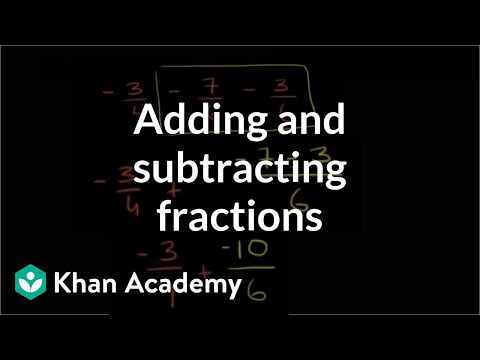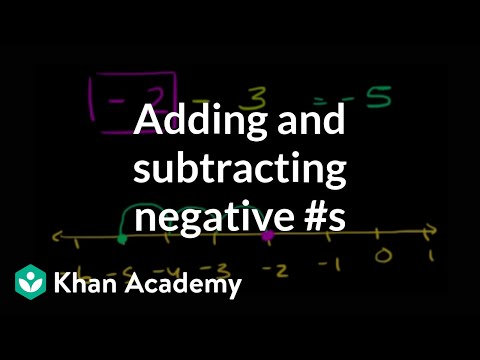Video

# Addition & subtraction: find the missing value (part 1 of 2) (Full video)

Khan Academy

## Supporting grades: 7

Description: Sal solves some missing value problems: -1 = 7 - __ and -10 + __ = -5 and 4 = -2 + __ and 6 + __ = -8. What I hope to do in this video is go through a bunch of examples that will hopefully give us an even better understanding of what it means to add or subtract a positive or a negative number. If I were to say that negative one is equal to seven minus blank, what should be in that blank? So let me put a number line right over here.

You must log inorsign upif you want to:*

*Teacher Advisor is 100% free.

### Other videos you might be interested in### Adding & subtracting fractions (Full video)

#### Khan Academy